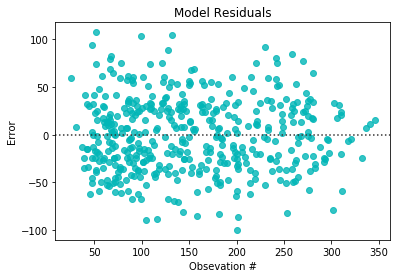01 Aug

## Plotting Residuals

Plotting you residuals for regression problems is crazy useful. This is another diagnostic plot that you can use to figure out if you did something incorrectly. Your residuals should look random, if they are not, you probably have an error in your model somewhere. I use the Seaborn residplot to plot all my residuals, the plot works really well with Scikit Learn models and Numpy arrays making it flexible.

### Import Preliminaries¶

In :
%matplotlib inline
%config InlineBackend.figure_format='retina'

# Import modulse
import matplotlib.pyplot as plt
import seaborn as sns

from sklearn import datasets
from sklearn.linear_model import LinearRegression

# Assigning targed and feature data
X = diabetes.data
y = diabetes.target

# Calling and training the linear model
reg = LinearRegression()
reg.fit(X,y)

# Predicteing target values with trained model
pred_y = reg.predict(X)


### Plot Residuals¶

In :
# Plotting the residuals of y and pred_y
sns.residplot(y,pred_y, color='#01B6B7')
plt.title('Model Residuals')
plt.xlabel('Obsevation #')
plt.ylabel('Error');Author: Kavi Sekhon